# Pandas : Convert Data frame index into column using dataframe.reset_index() in python

In this article, we will be exploring ways to convert indexes of a data frame or a multi-index data frame into its a column.

There is a function provided in the Pandas Data frame class to reset the indexes of the data frame.

## Dataframe.reset_index()

DataFrame.reset_index(self, level=None, drop=False, inplace=False, col_level=0, col_fill='')

It returns a data frame with the new index after resetting the indexes of the data frame.

• level: By default, reset_index() resets all the indexes of the data frame. In the case of a multi-index dataframe, if we want to reset some specific indexes, then we can specify it as int, str, or list of str, i.e., index names.
• Drop: If False, then converts the index to a column else removes the index from the dataframe.
• Inplace: If true, it modifies the data frame in place.

Let’s use this function to convert the indexes of dataframe to columns.

The first and the foremost thing we will do is to create a dataframe and initialize it’s index.

Code:
empoyees = [(11, ‘jack’, 34, ‘Sydney’, 70000) ,
(12, ‘Riti’, 31, ‘Delhi’ , 77000) ,
(13, ‘Aadi’, 16, ‘Mumbai’, 81000) ,
(14, ‘Mohit’, 31,‘Delhi’ , 90000) ,
(15, ‘Veena’, 12, ‘Delhi’ , 91000) ,
(16, ‘Shaunak’, 35, ‘Mumbai’, 75000 ),
(17, ‘Shaun’, 35, ‘Colombo’, 63000)]
# Create a DataFrame object
empDfObj = pd.DataFrame(empoyees, columns=[‘ID’ , ‘Name’, ‘Age’, ‘City’, ‘Salary’])
# Set ‘ID’ as the index of the dataframe
empDfObj.set_index(‘ID’, inplace=True)
print(empDfObj)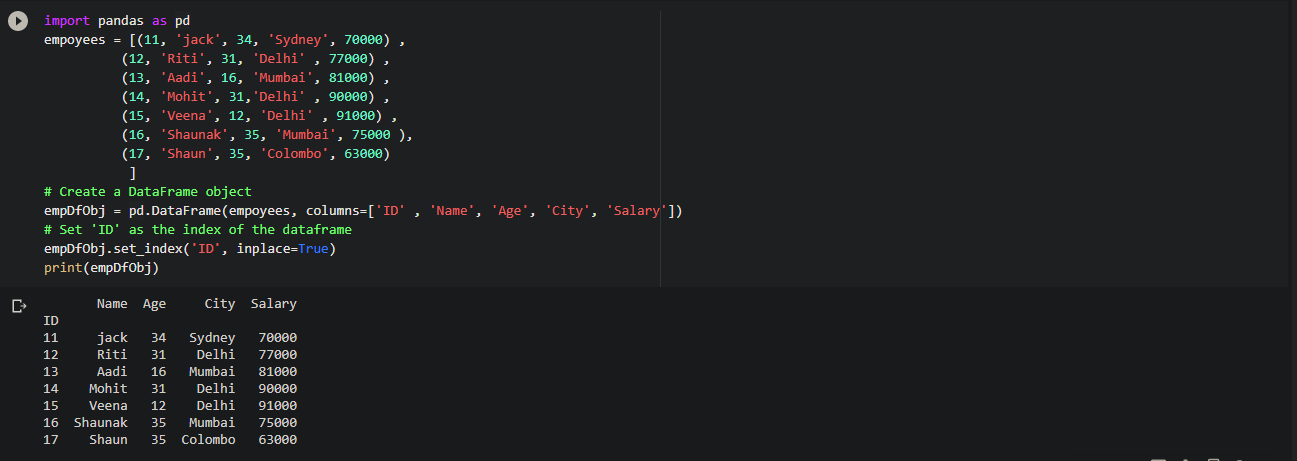Now, we will try different things with this dataframe.

### Convert index of a Dataframe into a column of dataframe

To convert the index ‘ID‘ of the dataframe empDfObj into a column, call the reset_index() function on that dataframe,

Code:
modified = empDfObj.reset_index()
print(“Modified Dataframe : “)
print(modified)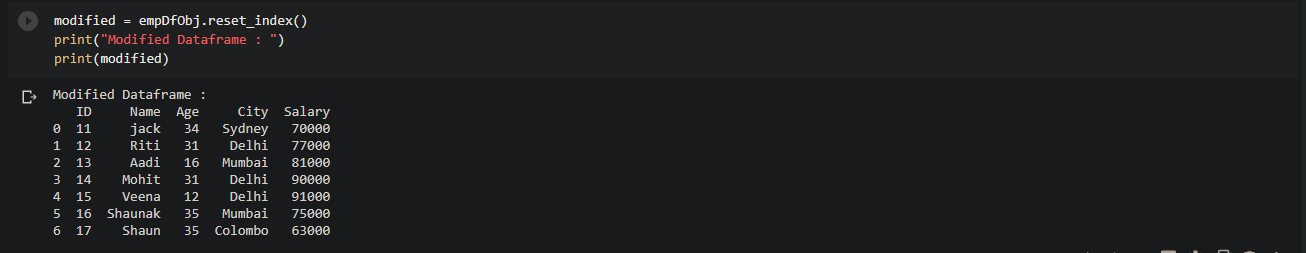Since we haven’t provided the inplace argument, so by default it returned the modified copy of a dataframe.

In which the indexID is converted into a column named ‘ID’ and automatically the new index is assigned to it.

Now, we will pass the inplace argument as True to proceed with the process.

Code:
empDfObj.reset_index(inplace=True)
print(empDfObj)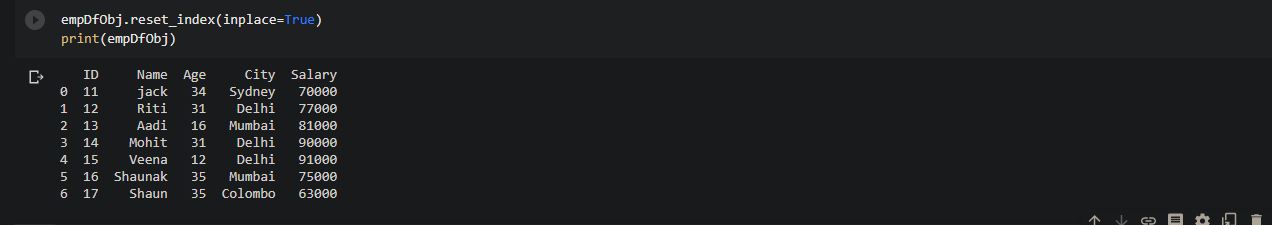Now, we will set the column’ID’ as the index of the dataframe.

Code:
empDfObj.set_index('ID', inplace=True)

### Remove index  of dataframe instead of converting into column

Previously, what we have done is convert the index of the dataframe into the column of the dataframe but now we want to just remove it. We can do that by passing drop argument as True in the reset_index() function,

Code:
modified = empDfObj.reset_index(drop=True)
print("Modified Dataframe : ")
print(modified)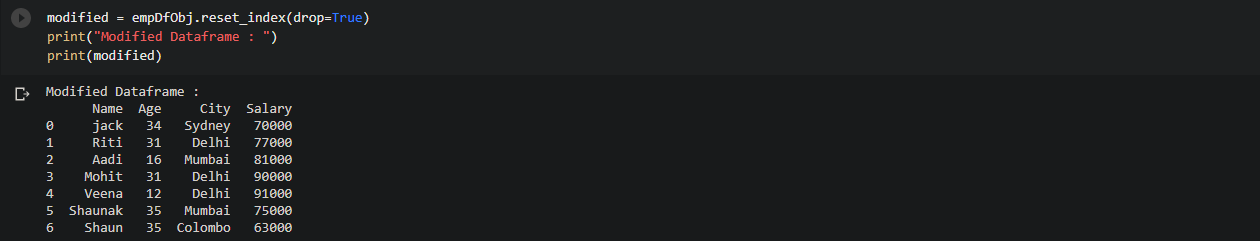We can see that it removed the dataframe index.

### Resetting indexes of a Multi-Index Dataframe

Let’s convert the dataframe object empDfObj  into a multi-index dataframe with two indexes i.e. ID & Name.

Code:
empDfObj = pd.DataFrame(empoyees, columns=['ID', 'Name', 'Age', 'City', 'Salary'])
# set multiple columns as the index of the the dataframe to
# make it multi-index dataframe.
empDfObj.set_index(['ID', 'Name'], inplace=True)
print(empDfObj)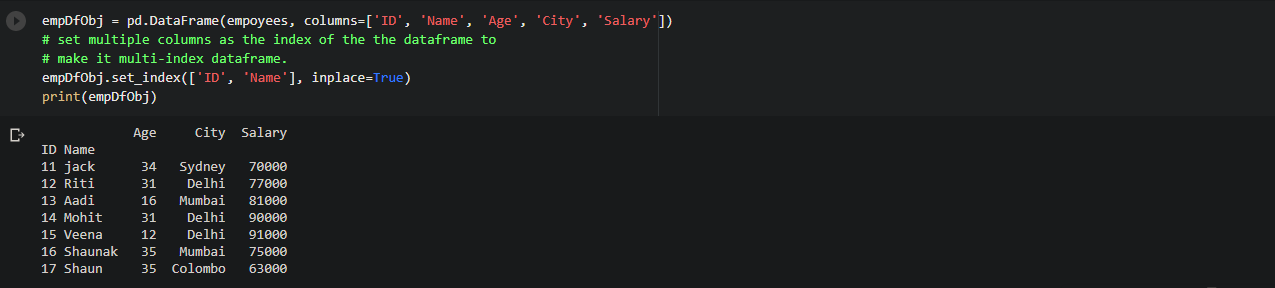### Convert all the indexes of Multi-index Dataframe to the columns of Dataframe

In the previous module, we have made a dataframe with the multi-index but now here we will convert the indexes of multi-index dataframe to the columns of the dataframe.

To do this, all we have to do is just call the reset_index() on the dataframe object.

Code:
modified = empDfObj.reset_index()
print(modified)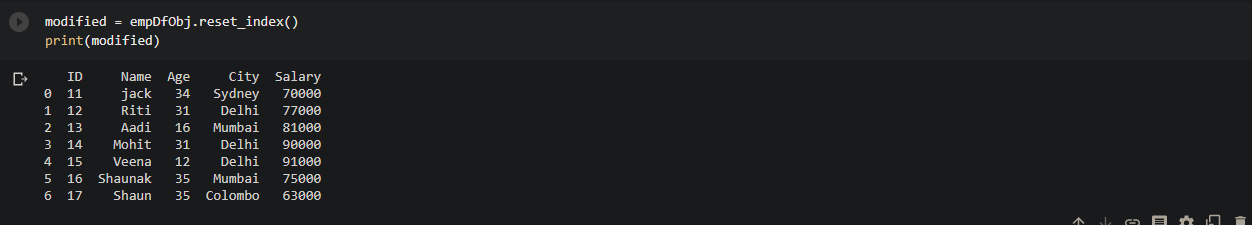It converted the index ID and Name to the column of the same name.

Suppose, we want to convert only one index from the multiple indexes. We can do that by passing a single parameter in the level argument.

Code:
modified = empDfObj.reset_index(level='ID')
print("Modified Dataframe: ")
print(modified)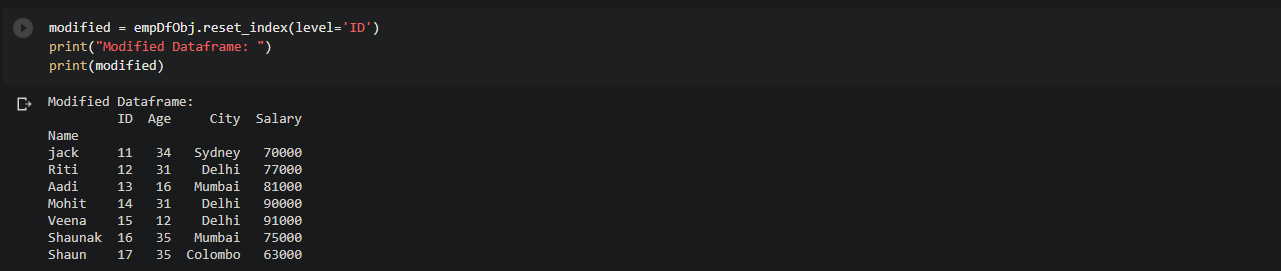It converted the index’ID’ to the column with the same index name. Similarly, we can follow this same procedure to carry out the task for converting the name index to the column.

You should try converting the code for changing Name index to column.

We can change both the indexes and make them columns by passing mutiple arguments in the level  parameter.

Code:
modified = empDfObj.reset_index(level=['ID', 'Name'])
print("Modified Dataframe: ")
print(modified)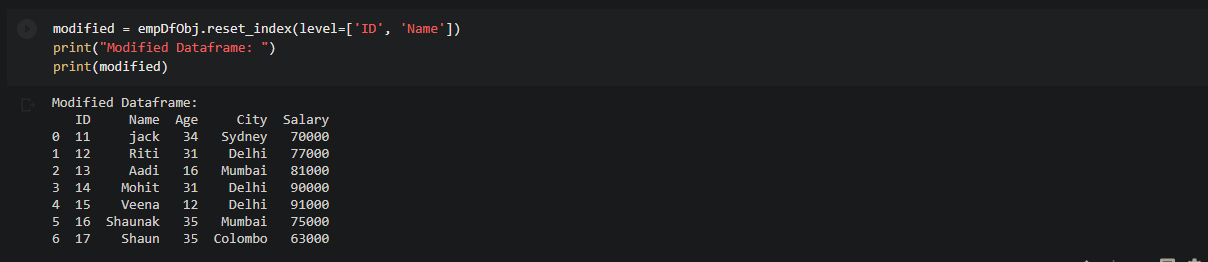The complete code:

import pandas as pd

def main():
# List of Tuples
empoyees = [(11, 'jack', 34, 'Sydney', 70000) ,
(12, 'Riti', 31, 'Delhi' , 77000) ,
(13, 'Aadi', 16, 'Mumbai', 81000) ,
(14, 'Mohit', 31,'Delhi' , 90000) ,
(15, 'Veena', 12, 'Delhi' , 91000) ,
(16, 'Shaunak', 35, 'Mumbai', 75000 ),
(17, 'Shaun', 35, 'Colombo', 63000)]
# Create a DataFrame object
empDfObj = pd.DataFrame(empoyees, columns=['ID' , 'Name', 'Age', 'City', 'Salary'])
# Set 'ID' as the index of the dataframe
empDfObj.set_index('ID', inplace=True)
print("Contents of the Dataframe : ")
print(empDfObj)
print('Convert the index of Dataframe to the column')
# Reset the index of dataframe
modified = empDfObj.reset_index()
print("Modified Dataframe : ")
print(modified)
print('Convert the index of Dataframe to the column - in place ')
empDfObj.reset_index(inplace=True)
print("Contents of the Dataframe : ")
print(empDfObj)
# Set 'ID' as the index of the dataframe
empDfObj.set_index('ID', inplace=True)
print('Remove the index of Dataframe to the column')
# Remove index ID instead of converting into a column
modified = empDfObj.reset_index(drop=True)
print("Modified Dataframe : ")
print(modified)
print('Reseting indexes of a Multi-Index Dataframe')
# Create a DataFrame object
empDfObj = pd.DataFrame(empoyees, columns=['ID', 'Name', 'Age', 'City', 'Salary'])
# set multiple columns as the index of the the dataframe to
# make it multi-index dataframe.
empDfObj.set_index(['ID', 'Name'], inplace=True)
print("Contents of the Multi-Index Dataframe : ")
print(empDfObj)
print('Convert all the indexes of Multi-index Dataframe to the columns of Dataframe')
# Reset all indexes of a multi-index dataframe
modified = empDfObj.reset_index()
print("Modified Mult-Index Datafrme : ")
print(modified)
print("Contents of the original Multi-Index Dataframe : ")
print(empDfObj)
modified = empDfObj.reset_index(level='ID')
print("Modified Dataframe: ")
print(modified)
modified = empDfObj.reset_index(level='Name')
print("Modified Dataframe: ")
print(modified)
modified = empDfObj.reset_index(level=['ID', 'Name'])
print("Modified Dataframe: ")
print(modified)
if __name__ == '__main__':
main()

Hope this article was useful for you and you grabbed the knowledge from it.

Want to expert in the python programming language? Exploring Python Data Analysis using Pandas tutorial changes your knowledge from basic to advance level in python concepts.

Read more Articles on Python Data Analysis Using Padas – Modify a Dataframe